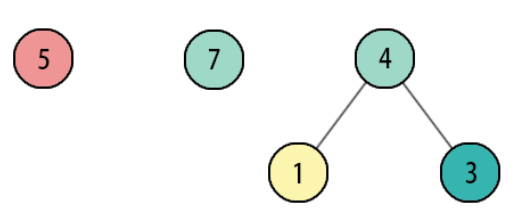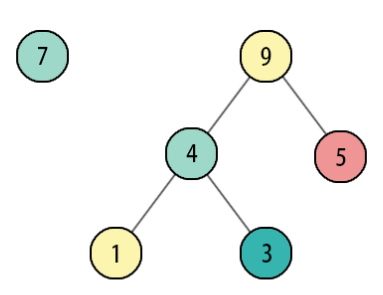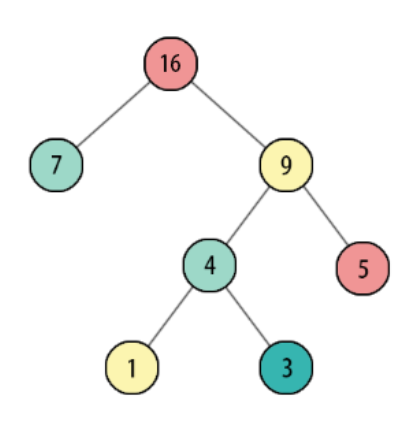• 哈夫曼树是一种用来对字符进行编码的数据结构，可以根据字符的使用频率来决定字符的二进制表示，使得转化后的二进制序列尽可能短。 哈夫曼树的具体介绍见此博客用漫画介绍哈弗曼树 接下来介绍哈夫曼树的构造方法，...

简介

哈夫曼树是一种用来对字符进行编码的数据结构，可以根据字符的使用频率来决定字符的二进制表示，使得转化后的二进制序列尽可能短。
哈夫曼树的具体介绍见此博客用漫画介绍哈弗曼树

接下来介绍哈夫曼树的构造方法，假设给定n个字符及其对应的频率（出现次数），求出用哈夫曼编码后编码串的长度。
比如：

4 //字符个数
a 1
b 2
c 3
d 4

会返回19。（如果不知道怎么算请看上面链接的博客）

c++构造

方法一

首先可以想到用优先队列，将包含频率信息的节点加入队列，然后每次从队列中拿出两个最小的节点a和b，求出频率和，构造新的节点，其左右子节点分别指向a和b，将新节点加入队列。重复此过程直至队列中只剩一个节点。
在上面的步骤中我们模拟哈夫曼树的构造过程，确确实实地构造出一棵树来，接下来只需要由这棵树的根节点开始遍历，找到每个叶子节点，将它们的值（即频率）和深度（即编码长度）的乘积加起来，就是我们要的结果。
时间复杂度：O(nlogn)
ps:这个代码没有释放指针，但是这不是要点，所以忽略了。

#include<iostream>
#include<queue>
#include<unordered_map>
#include <cstdio>
#include <cstring>
#include <algorithm>
#include <vector>
using namespace std;
struct Node {
long long val;
Node* left, *right;
Node(long long val) {
this->val = val;
left = NULL;
right = NULL;
}
};

struct cmp {
bool operator()(const Node* a, const Node* b) const{
return a->val > b->val;
}
};

long long search(Node* root, long long level) {
if (root == NULL) return 0;
if (root->left == NULL && root->right == NULL) return root->val * level;
return search(root->left, level + 1) + search(root->right, level + 1);
}
int main() {
long long n;
cin >> n;
if (n == 0) {
cout << 0;
return 0;
}
long long arr[n];
char c;
for (long long i = 0; i < n; ++i) {
cin >> c >> arr[i];
}
if (n == 1) {
cout << arr;
return 0;
}
priority_queue<Node*, vector<Node*>, cmp> pq;
for (long long i = 0; i < n; ++i) {
//if (arr[i] <= 0) continue;
Node* t = new Node(arr[i]);
pq.push(t);
}

if (pq.size() == 1) {
cout << pq.top()->val << endl;
return 0;
}
Node* root;
while(pq.size() > 1) {
Node* a = pq.top();
pq.pop();
Node* b = pq.top();
pq.pop();
Node* sum = new Node(a->val + b->val);
sum->left = a;
sum->right = b;
root = sum;
pq.push(sum);
}
cout << search(root, 0) << endl;
}

方法二

如果不想用优先队列，不想自己写比较函数，可以怎样写呢？
注意到一个规律：每次从队列中拿出最小的两个节点按值相加，得到的新节点的值是递增的，假设我们只用普通的数组来存储最初的节点，并且用另一个数组来存储新增的节点，再用两个指针分别指向两个数组的下标，通过一定的控制（从左边数组拿或从右边数组拿）可以实现。具体请看代码。

#include<cstring>
#include<algorithm>
#include<vector>
#include<iostream>
using namespace std;
int n;
vector<long long> a;
int idx;

inline long long popleft(int i) {
return a[i][idx[i]++];
}

inline long long getmin() {
if (idx >= (int)a.size()) {
return popleft(1);
}
if (idx >= (int)a.size()) {
return popleft(0);
}
if (a[idx] < a[idx]) {
return popleft(0);
}
return popleft(1);
}

int main() {
cin >> n;
if (n == 0) {
cout << 0 << endl;
return 0;
}
long long t1, t2;
char c;
for (int i = 0; i < n; ++i) {
cin >> c >> t2;
a.push_back(t2);
}
if (n == 1) {
cout << t2 << endl;
return 0;
}
sort(a.begin(), a.end());

long long ans = 0;
for (int i = 1; i < n; ++i) {
t1 = getmin();
t2 = getmin();
ans += t1 + t2;
a.push_back(t1 + t2);
}
cout << ans << endl;
return 0;
}

时间复杂度依然是O(nlogn）。

展开全文c++ 数据结构 算法 队列
• 给定n个权值作为n的叶子结点，构造一棵二叉树，若带权路径长度达到最小，称这样的二叉树为最优二叉树，也称为哈夫曼树(Huffman Tree)。哈夫曼树是带权路径长度最短...使用数组构建哈夫曼树，并可用该树构造哈夫曼编码。C++ 数据结构
• 实 验 报 告 实验目的 掌握哈夫曼树的基本概念及所用的存储结构 掌握哈夫曼树的建立算法 掌握哈夫曼树的应用哈夫曼树的编码和译码 实验内容 给定权值529781423311建立哈夫曼树输出哈夫曼编码对上述给定的哈夫曼树及...
• 本文以实例形式讲述了C++实现哈夫曼树简单创建与遍历的方法，比较经典的C++算法。 本例实现的功能为：给定n个带权的节点，如何构造一棵n个带有给定权值的叶节点的二叉树，使其带全路径长度WPL最小。 据此构造出最...
• 哈夫曼树 定义： 在一个二叉树中，若带权长度达到最小，则称这样的二叉树为最优二叉树，也称为哈夫曼树哈夫曼树的构造： 假设有n个权值，则构造出的哈夫曼树有n个叶子结点。n个权值分别设为 w1,w2,…,wn , 则...

哈夫曼树

定义： 在一个二叉树中，若带权长度达到最小，则称这样的二叉树为最优二叉树，也称为哈夫曼树。

哈夫曼树的构造：
假设有n个权值，则构造出的哈夫曼树有n个叶子结点。n个权值分别设为 w1,w2,…,wn , 则哈夫曼树的构造规则为：

1. 将 w1,w2,…,wn 看成有n棵树的森林。
2. 在森林中选出两个根节点的权值最小的树合并，作为一棵新的左、右子树，且新的树的根节点权值为其左右子树根节点权值之和。
3. 从森林中删除选取的两棵树，并将新树加入森林。
4. 重复步骤2，3直到森林只剩一棵树为止，该树即为所求得的哈夫曼树。

下面给出哈夫曼树的构造过程，假定给定的叶子节点的权分别为1，5，7，3，则构造哈夫曼树的过程如图

1.初始化森林2.一次合并后的森林3.二次合并后的森林4.三次合并后的森林C++实现代码

定义二叉树结点类

const int MAX=1000;//权重一般不会超过这个数，勉强把它当做无穷大
class btreenode
{
public:
int weight;//权重
btreenode *left,*right;//左右结点
};

定义二叉树类

class btree
{
public:
btreenode *root;
int capacity;
int *a;
void input()
{
cout<<"请输入要建立的树的结点个数";
cin>>capacity;
a=new int[capacity];
for(int i=0;i<capacity;i++)
{
cin>>a[i];
}
}
void build()
{
root=NULL;
int i;
int min=a;
int p;//存放找到的最小值位置
while(min<1000)
{
for(i=0,min=a,p=0;i<capacity;i++)
{
if(min>=a[i])
{
min=a[i];
p=i;
}
}//找出最小值
if(min<1000)
{
if(root==NULL)//如果哈夫曼树还没有结点，就产生第一个根节点
{
root=new btreenode;
root->weight=a[p];
root->left=NULL;
root->right=NULL;
a[p]=1000;
}
else//如果已经有结点就在把最小值放在现在根节点的兄弟位置
{
btreenode *t;
btreenode *q;
t=new btreenode;
q=new btreenode;

t->weight=a[p];
t->left=NULL;
t->right=NULL;//最小值的节点
a[p]=1000;
q->weight=root->weight+t->weight;//新的根节点，权重为下面两个权重之和
if(root->weight>t->weight)
{
q->left=t;
q->right=root;
root=q;
}
else
{
q->left=root;
q->right=t;
root=q;
}
}
}
}
}
};

代码如果有什么不足，请大佬指点，本人虚心学习

展开全文C++ 哈夫曼树
• 然后构建Huffman，得到每个字符对应的Huffman编码 字符串的每个字符串的huffman编码为： a 10 g 011 d 110 b 111 f 000 h 001 s 010 最后得到整个字符串的Huffman编码 整个字符串...

Huffman tree

//输出Huffman编码
本程序实现了如何将一串字符串输出为Huffman编码

VER       ||      1.0
DATE      ||      15/11/2017
AUTHER    ||      WUD

比输入字符串agdfaghdabsba

通过字符串的处理可以知道其中每个字符出现的次数

字符a出现了4次
字符g出现了2次
字符d出现了2次
字符b出现了2次
字符f出现了1次
字符h出现了1次
字符s出现了1次

然后构建Huffman树，得到每个字符对应的Huffman编码

字符串的每个字符串的huffman编码为：
a 10
g 011
d 110
b 111
f 000
h 001
s 010

最后得到整个字符串的Huffman编码

整个字符串的Huffman编码为：
10011110000100110011101011101011110

#include<iostream>
#include<string>
#include <windows.h>
#include<stdio.h>
using namespace std;

/*
--------------------------

VER       ||      1.0
DATE      ||      15/11/2017
AUTHER    ||      WUD

--------------------------
*/

struct huffTree{
int parent;
int lchild;
int rchild;
int weight;
string flag;
};

struct Lowest_Node{
char ch;
int ch_num;
};

void ShowProgram()
{
HANDLE handle = GetStdHandle(STD_OUTPUT_HANDLE);
SetConsoleTextAttribute(handle, FOREGROUND_INTENSITY | FOREGROUND_RED);
int j;
for(j=0; j<27; j++){
printf("-");
Sleep(1);}
printf("\n");
printf("VER           ||      1.0\n");
printf("DATE          ||      15/11/2017\n");
printf("AUTHER        ||      WUD\n");
for(j=0; j<27; j++){
printf("-");
Sleep(1);
}
printf("\n");
Sleep(200);
}

void coding(int length, huffTree tree[], int n, int &a, int &b){
int i;
int r,s;
r=s=length;
for(i=0;i<n;i++){
if((tree[i].weight<r)&&(tree[i].parent==-1)){
r=tree[i].weight;
a=i;
}
}
for(i=0;i<n;i++){
if((tree[i].weight<s)&&(i!=a)&&(tree[i].parent==-1)){
s=tree[i].weight;
b=i;
}
}
}

void frequency(string str){
int length = str.length();                  //长度
Lowest_Node *node=new Lowest_Node[length];  //声明最0节点
int i,j;            //循环因子
for(i=0;i<length;i++){
node[i].ch_num = 0;         //初始化频度
}
int char_type_num = 0;          //初始为0种字符
for(i=0;i<length;i++){          //循环整个字符串
for(j=0;j<char_type_num;j++){
if(str[i]==node[j].ch||(node[j].ch>='a'&&node[j].ch<='z'&&str[i]+32==node[j].ch))
break;}
if(j<char_type_num){node[j].ch_num++;}
else{
if(str[i]>='A'&& str[i]<='Z')
node[j].ch=str[i]+32;
else node[j].ch = str[i];
node[j].ch_num++;
char_type_num++;
}
}

//按频度从大到小排序
for(i=0;i<char_type_num;i++){
for(j=i;j<char_type_num;j++){
if(node[j].ch_num<node[j+1].ch_num){//如果前一个小于后一个，交换
int temp;                       //临时频度
char ch_temp;                   //临时字符
temp=node[j].ch_num;
ch_temp=node[j].ch;
node[j].ch_num=node[j+1].ch_num;
node[j].ch=node[j+1].ch;
node[j+1].ch_num=temp;
node[j+1].ch=ch_temp;
}
}
}

for(i=0;i<char_type_num;i++){               //打印字符频度
cout<<"字符"<<node[i].ch<<"出现了"<<node[i].ch_num<<"次"<<endl;}
huffTree *huff = new huffTree[2*char_type_num-1];//声明需位于确定char_type_num值后
huffTree temp;
string *code = new string[2*char_type_num-1];
for(i=0;i<2*char_type_num-1;i++){             //节点初始化
huff[i].parent=-1;
huff[i].lchild=-1;
huff[i].rchild=-1;
huff[i].flag=-1;
}
for(j=0;j<char_type_num;j++){               //将排序后的第0级节点权重赋值给树节点
huff[j].weight = node[j].ch_num;
}
int min1,min2;
for(int k=char_type_num;k<2*char_type_num-1;k++){       //赋值0级以上的节点
coding(length,huff,k,min1,min2);
huff[min1].parent=k;
huff[min2].parent=k;
huff[min1].flag="0";
huff[min2].flag="1";
huff[k].lchild=min1;
huff[k].rchild=min2;
huff[k].weight=huff[min1].weight+huff[min2].weight;
}
for(i=0;i<char_type_num;i++){
temp = huff[i];
while(1){
code[i]=temp.flag+code[i];
temp =huff[temp.parent];
if(temp.parent==-1)break;
}
}
cout<<"字符串的每个字符串的huffman编码为："<<endl;
for(i=0;i<char_type_num;i++){
cout<<node[i].ch<<" "<<code[i]<<endl;
}
cout<<"整个字符串的Huffman编码为："<<endl;
for(i=0;i<length;i++){
for(j=0;j<char_type_num;j++){
if(str[i]==node[j].ch)
cout<<code[j];
}
}
//释放内存
delete[] node;
node=NULL;
delete[] huff;
huff=NULL;
delete[] code;
code = NULL;
}

int main(){
ShowProgram();
int length=0;               //字符串长度；
string str;                 //目标字符串；
cout<<"请输入字符串：";
cin>>str;
frequency(str);              //求各个字符串的频度

return 0 ;
}

展开全文c语言 编码
• 哈夫曼树-----即最优二叉树，带权路径长度（WPL）最小的二叉树，经常应用于数据压缩。 对于普通需要将字符串编码传输时，普通的编码需要将每个字符编程定长的二进制格式，但是每个字符在数据中通常出现的概率不同的...

介绍

哈夫曼树-----即最优二叉树，带权路径长度（WPL）最小的二叉树，经常应用于数据压缩。
对于普通需要将字符串编码传输时，普通的编码需要将每个字符编程定长的二进制格式，但是每个字符在数据中通常出现的概率不同的，所以，需要使用不定长的二进制表示是编码的表示更短。

前缀编码：对于每个字符设计长度不等的编码，必须使任一字符的编码都不是另一个字符的前缀。这样才能让编码不出现二义性。
Huffman编码：是前缀编码，也是最短编码—>压缩率最高。且是无损压缩！！！

连续存储方式建立Huffman树

//树结点结构
struct  Node
{
double weight; //权重
int parent,lson,rson;   //父结点、左孩子、右孩子下标
};

//初始化
void init(vector<double>& nums,Node* huff)
{
int n=nums.size();
//从下标1开始
for(int i=1;i<=n;i++){
huff[i].parent=0;
huff[i].lson=0;
huff[i].rson=0;
huff[i].weight=nums[i-1];
}
}
//从当前所有父结点为0的节点中选择权重最小的两个结点A，B
vector<int> select(Node* huff,int len)
{
int pA=0,pB=0;
double MinA=INT32_MAX,MinB=INT32_MAX;

for(int i=1;i<len;i++){
if(!huff[i].parent && huff[i].weight){
if(MinA>huff[i].weight){
pB=pA;pA=i;
MinB=MinA;MinA=huff[i].weight;
}
else if(MinB>huff[i].weight){
pB=i;MinB=huff[i].weight;
}
}
}
return {pA,pB}; //返回对应结点的下标
}

//哈夫曼树共有2*n-1个结点
//前面n个结点是叶子结点，后面n-1个节点是构造出来的父节点
Node* build(vector<double>& nums)
{
int n=nums.size();
Node* huffTr=new Node[2*n];

init(nums,huffTr);  //初始化---1、构造森林全是根

for(int i=n+1;i<=2*n-1;i++){

//从当前所有父结点为0的节点中选择权重最小的两个结点A，B---2、选择两小造新树
vector<int> ans=select(huffTr,2*n);
int j=ans,k=ans;

//生成新的结点，并把该节点的左右子树设置A，B---3、删除两小添新人
huffTr[i].weight=huffTr[j].weight+huffTr[k].weight;
huffTr[i].parent=0;
huffTr[i].lson=j;
huffTr[i].rson=k;

//设置A，B的父结点
huffTr[j].parent=i;
huffTr[k].parent=i;
//---4、重复2、3剩单根
}
return huffTr;
}

建树与编码的测试代码int main()
{
//测试用例1：
//A、B、C、D、E、F、G字符对应的概率（即权重）用数组nums表示
vector<double> nums={0.4,0.3,0.15,0.05,0.04,0.03,0.03};
//A,B,C,D,E,F,G
int n=nums.size();

auto huff=build(nums);  //构建Huffman树
//遍历
for(int i=1;i<=2*n-1;i++){
cout<<huff[i].weight<<" "<<huff[i].parent<<" "<<huff[i].lson<<" "<<huff[i].rson<<endl;
}
cout<<endl;

//测试用例2：
//将ABCDEFG字符串进行Huffman编码，从底向上遍历
vector<string> res;
for(int i=1;i<=7;i++){
string a;
int cur=i;
//遍历到根节点时退出循环（根节点的parent为0），说明该字符的编码已完成
while(huff[cur].parent!=0){
int par=huff[cur].parent;
//当前结点是其父结点的左孩子，则标0;是右孩子则标1
if(huff[par].lson==cur){
a='0'+a;
}else{
a='1'+a;
}
cur=par;
}
res.emplace_back(a);
}
//打印每个字符的编码
for(int i=1;i<=7;i++){
char c=i-1+'a';
cout<<c<<" :"<<res[i-1]<<endl;
}

return 0;
}

展开全文c++ 开发语言
• 哈夫曼树C++实现

千次阅读 2020-07-06 22:58:24
哈夫曼树 给定一组具有确定权值的叶子结点，可以造出不同的二叉树，将其中带权路径长度最小的二叉树称为哈夫曼树（Huffman tree）。 哈夫曼节点类 哈夫曼节点会存储节点的权重以及，ch是节点对应的编码字符的下标...数据结构 C++
• 1、输入结点个数和权重创建哈夫曼树 #define _CRT_SECURE_NO_WARNINGS #include<bits/stdc++.h> using namespace std; //haffman 树的结构 typedef struct { //叶子结点权值 unsigned int weight; //指向...c++ 数据结构 leetcode
• 信息论课程设计-哈夫曼编码。将英文字符的统计概率作为待编码节点权值。编程得出哈夫曼的码表；输入一段英文字符，利用码表对其编码、译码。显示整个流程哈夫曼编码
• 给定n个权值作为n个叶子结点，构造一棵二叉树，若该树的带权路径长度达到最小，称这样的二叉树为最优二叉树，也称为哈夫曼树(Huffman Tree)。...本资源通过传入数组的方式构建哈夫曼树，并实现构建后哈夫曼树的遍历
• C++版全网最全、详解、引导思考的哈夫曼树和哈夫曼编码讲解，剖析了关键代码，适合收藏~算法 数据结构 c++ 树结构
• c++代码实现哈夫曼树的创建、编码以及求WPL (顺序结构) 文章目录 文章目录c++代码实现哈夫曼树的创建、编码以及求WPL (顺序结构)exercise problemcoderunning resultsummary exercise problem ​ 构造哈夫曼树生产...数据结构 二叉树 算法 c++
• 输入字符个数，权值，打印哈夫曼树，编码和译码，源代码，可以用c-free运行的那种，求各位大神帮忙解答，要完整的代码拜托了c++
• C++ 实现哈夫曼树哈夫曼树的定义 哈夫曼树的定义   将树中的结点赋予一个有某种意义的数值，称此数值为该结点的权。从树根结点到该结点之间的路径长度与该结点上权的乘积称为结点的带权路径长度。树中所有叶子结点...
• 主要为大家详细介绍了C语言实现哈夫曼树构建，文中示例代码介绍的非常详细，具有一定的参考价值，感兴趣的小伙伴们可以参考一下C语言 哈夫曼树
• c++编写的哈夫曼树的建立过程,构造函数，输出方法等
• 给定N个权值作为N个叶子结点，构造一棵二叉树，若该树的带权路径长度达到最小，称这样的二叉树为最优二叉树，也称为哈夫曼树(Huffman Tree)。 哈夫曼树是带权路径长度最短的树，权值较大的结点离根较近。 步骤 求{11...c++
• C++实现哈夫曼树与哈夫曼编码

千次阅读 多人点赞 2019-06-02 09:51:08
哈夫曼树的存储表示 typedef char ElemType; typedef struct { ElemType data; //结点存的数据 int weight; //结点的权值 int parent,lchild,rchild; //结点的双亲、左孩子、右孩子的下标 } HTNode,*HuffmanT...哈夫曼树 哈夫曼编码
• C/C++哈夫曼树的构造、编码以及译码

万次阅读 多人点赞 2017-01-05 15:10:34
题目：假设用于通信的...要求：画出哈夫曼树哈夫曼树的构造 哈夫曼编码及译码的实现 我从课本上面摘抄了一个题目，题目大概是上面这样的，我们这里只是详细的说明一下哈弗曼树要怎么构建。借用一下这个题目。哈夫曼
• 自己实现的哈夫曼树，代码不超过100行，用到了优先队列
• // 用最小栈方式构建哈弗曼树// 定义一个哈夫曼树的节点struct MinHeapNode { // One of the input characters char data; // Frequency of the character unsigned freq; // 哈夫曼的编码值, #号表示无编码 ...数据结构 c 哈弗曼树 快速
• 本程序的主要数据结构在于构建哈夫曼树，然而采用链表的形式的话增加后续的查找，递归遍历等操作的复杂度，故在此选用结构数组的形式来存储哈夫曼树，这会导致后续的许多算法理解起来相较于链...c++ 开发语言 后端
• 用C语言实现哈夫曼树和生成哈夫曼编码，首先生成哈夫曼树哈夫曼树是从中选取权值最小的两个进行相加，将这两个分别做为生成的左子树和右子树，左子树要比右子树小。然后将相加得到的值从新放入，然后又从中找到...C/C++ 数据结构 哈夫曼树 哈夫曼编码
• 哈夫曼树又称作最优二叉树，是带权路径长度最小的二叉树。 一、算法步骤： 构造哈夫曼树算法的实现可以分成两大部分。 ①初始化:首先动态申请2n个单元;然后循环2n-1次，从1号单元开始，依次将1至m所有单元中的...c++ 数据结构...

c++构建哈夫曼树c++ 订阅